Question

The decomposition reaction of A to B has a rate constant of 1.49×10-4 s-1:

A → 2B

How long will it take for the concentration of A to decrease by 18.7%? (answers given in minutes)

a)93.6

b)48.2

c) 33.3

d) 97.8

e) 23.1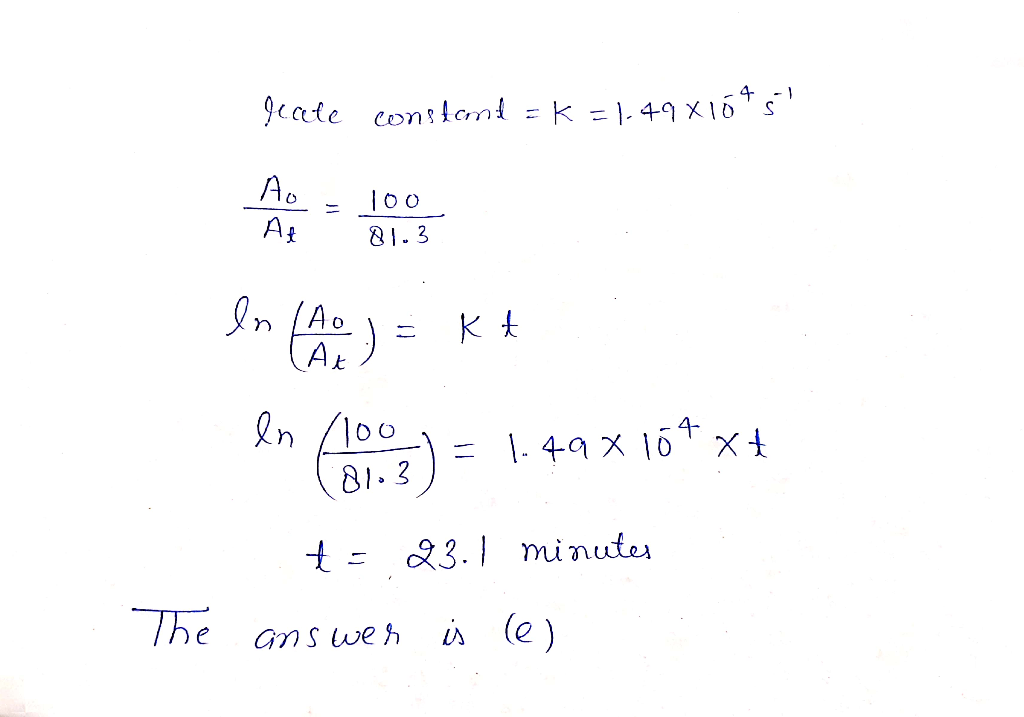#### Earn Coins

Coins can be redeemed for fabulous gifts.

Similar Homework Help Questions
• ### The decomposition of XY is second order in XYand has a rate constant of 7.12×10−3 M−1⋅s−1...

The decomposition of XY is second order in XYand has a rate constant of 7.12×10−3 M−1⋅s−1 at a certain temperature. What is the half-life for this reaction at an initial concentration of 0.100 M? How long will it take for the concentration of XY to decrease to 12.5% of its initial concentration when the initial concentration is 0.100 M? How long will it take for the concentration of XY to decrease to 12.5% of its initial concentration when the initial...

• ### The decomposition of SO2Cl2 is first order in SO2Cl2 and has a rate constant of 1.48×10−4...

The decomposition of SO2Cl2 is first order in SO2Cl2 and has a rate constant of 1.48×10−4 s−1 at a certain temperature. How long will it take for the concentration of SO2Cl2 to decrease to 25% of its initial concentration? If the initial concentration of SO2Cl2 is 1.00 M, how long will it take for the concentration to decrease to 0.76 M ? If the initial concentration of SO2Cl2 is 0.175 M , what is the concentration of SO2Cl2 after 210...

• ### The decomposition of SO2Cl2 is first order in SO2Cl2 and has a rate constant of 1.44×10−4...

The decomposition of SO2Cl2 is first order in SO2Cl2 and has a rate constant of 1.44×10−4 s−1 at a certain temperature. A) How long will it take for the concentration of SO2Cl2 to decrease to 25% of its initial concentration? Express your answer using two significant figures. B)If the initial concentration of SO2Cl2 is 1.00 M, how long will it take for the concentration to decrease to 0.74 M ? Express your answer using two significant figures. Please explain step...

• ### The decomposition of SO2Cl2 is first order in SO2Cl2 and has a rate constant of 1.36×10−4...

The decomposition of SO2Cl2 is first order in SO2Cl2 and has a rate constant of 1.36×10−4 s−1 at a certain temperature. Part A: If the initial concentration of SO2Cl2 is 1.00 M, how long will it take for the concentration to decrease to 0.78 M ? Part B: If the initial concentration of SO2Cl2 is 0.125 M , what is the concentration of SO2Cl2 after 180 s? Part C: If the initial concentration of SO2Cl2 is 0.125 M , what...

• ### The decomposition of SO2Cl2 is first order in SO2Cl2 and has a rate constant of 1.42*10^-4...

The decomposition of SO2Cl2 is first order in SO2Cl2 and has a rate constant of 1.42*10^-4 1/s at a certain temperature. How long will it take for the reaction to be 75.0% complete?

• ### 6. The first-order decomposition of H:O2 at -30 °C occurs with a rate constant of 0.0165...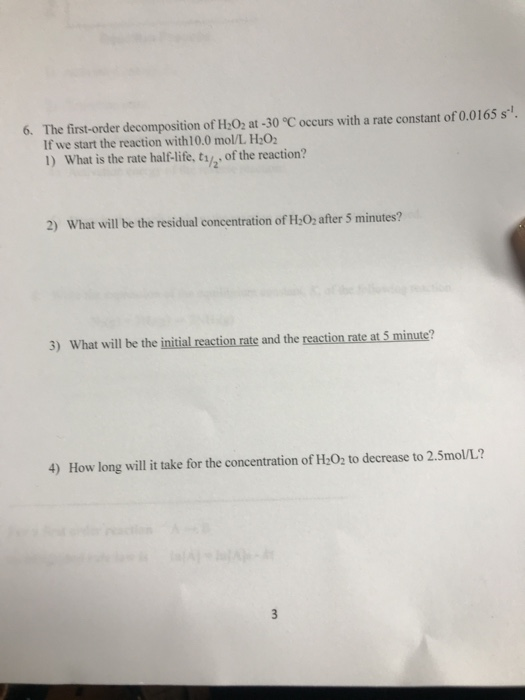6. The first-order decomposition of H:O2 at -30 °C occurs with a rate constant of 0.0165 s. If we start the reaction with10.0 mol/L H:0 1) What is the rate half-life, ty2 of the reaction? 2) What will be the residual concentration of H O, after 5 minutes? 3) What will be the initial reaction rate and the reaction rate at 5 minute? 4) How long will it take for the concentration of H 02 to decrease to 2.5mo/L?

• ### A zero-order reaction A - B, has a rate constant of 0.725 M. s 1. How...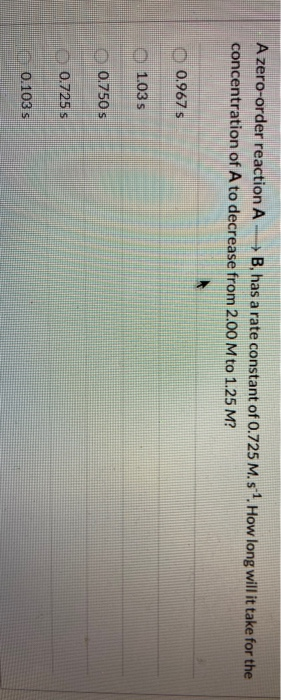A zero-order reaction A - B, has a rate constant of 0.725 M. s 1. How long will it take for the concentration of A to decrease from 2.00 M to 1.25 M? 0.967 s 1.03 s 0.750 s 0.725 s 0.103 s

• ### The decomposition of XY is second order in XY and has a rate constant of 6.98×10−3...

The decomposition of XY is second order in XY and has a rate constant of 6.98×10−3 L mol−1 s−1 at a certain temperature. Part B If the initial concentration of XY is 0.150 mol L−1 , how long will it take for the concentration to decrease to 6.60×10−2 mol L−1 ?   Express your answer using two significant figures. t= _______ s Part C If the initial concentration of XY is 0.050 mol L−1, what is the concentration of XY after...

• ### The decomposition of SO2Cl2 is first order in SO, Cl, and has a rate constant of...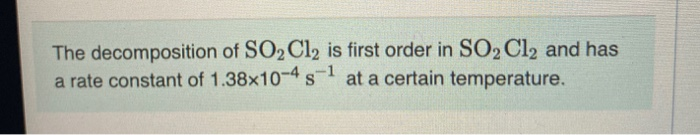The decomposition of SO2Cl2 is first order in SO, Cl, and has a rate constant of 1.38x10-4 5-1 at a certain temperature. Part A What is the half-life for this reaction? ΟΙ ΑΣφ ? 11/2= Submit Request Answer Part B How long will it take for the concentration of SO, Cl, to decrease to 25% of its initial concentration? Express your answer using two significant figures. ΟΙ ΑΣΦ ? Part C If the initial concentration of SO, Cl, is 1.00...

• ### The decomposition of SO,Cl, is first order in SO, Cl, and has a rate constant of...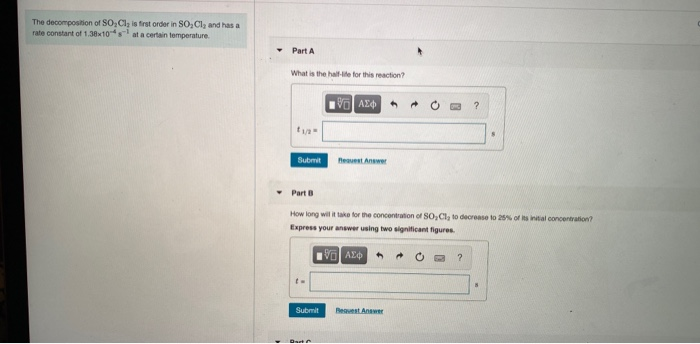The decomposition of SO,Cl, is first order in SO, Cl, and has a rate constant of 1.38x10 - at a certain temperature Part A What is the half-life for this reaction? 1 | ΑΣφ και ? \$ Submit Het we Part How long wil it take for the concentration of SO,Cl to decrease to 25% of its initial concentration? Express your answer using two significant figures. VE ΑΣφ ? Submit Beavest A The decomposition of SO,Cl is first order in...